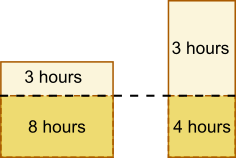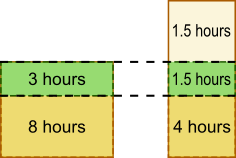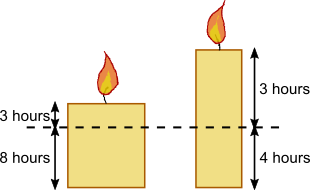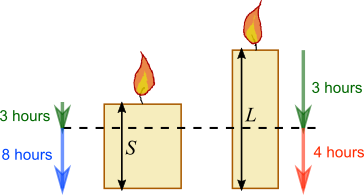#### You may also like### Building Tetrahedra

Can you make a tetrahedron whose faces all have the same perimeter?A 1 metre cube has one face on the ground and one face against a wall. A 4 metre ladder leans against the wall and just touches the cube. How high is the top of the ladder above the ground?Four rods are hinged at their ends to form a convex quadrilateral. Investigate the different shapes that the quadrilateral can take. Be patient this problem may be slow to load.

# Candles

##### Age 14 to 16 Short Challenge Level:

Using an area model
Let the height represent the height and the area the time left, so width is thickness (or other variable that affects the rate of the burning of the candle).After 3 hours, 8 hours left and 4 hours left

$\therefore$ width of shorter candle is $2\times$ width of longer candle$\therefore$ 3 hours on the short candle has the same height as 1.5 hours on the long candle

$\therefore$ heights are in the ratio (4 + 1.5) : (4 + 1.5 + 1.5)
5.5 : 7
11 : 14

Using ratesAfter 3 hours, both candles are the same length.

This will burn for 8 hours on the shorter candle and 4 hours on the longer candle.

$\therefore$ the longer candle burns twice as fast as the shorter candle.

So if the longer candle were as fat (or whatever other property affects burning time) as the shorter candle, it would burn for 7$\times$2 = 14 hours.

So their lengths are in the ratio 14 : 11.

Using fractionsAfter 3 hours:
shorter candle length is $\frac8{11}S$
longer candle length is $\frac47L$

So $\frac8{11}S=\frac47L\Rightarrow7\times8\times S=11\times4\times L\Rightarrow14\times S=11\times L$.

This means the ratio $L:S$ is $14:11$.

(This follows because if $L=14k$ and $S=11k$, then $14\times S=14\times11k$ and $11\times L=11\times14k$, which are equal).

You can find more short problems, arranged by curriculum topic, in our short problems collection.